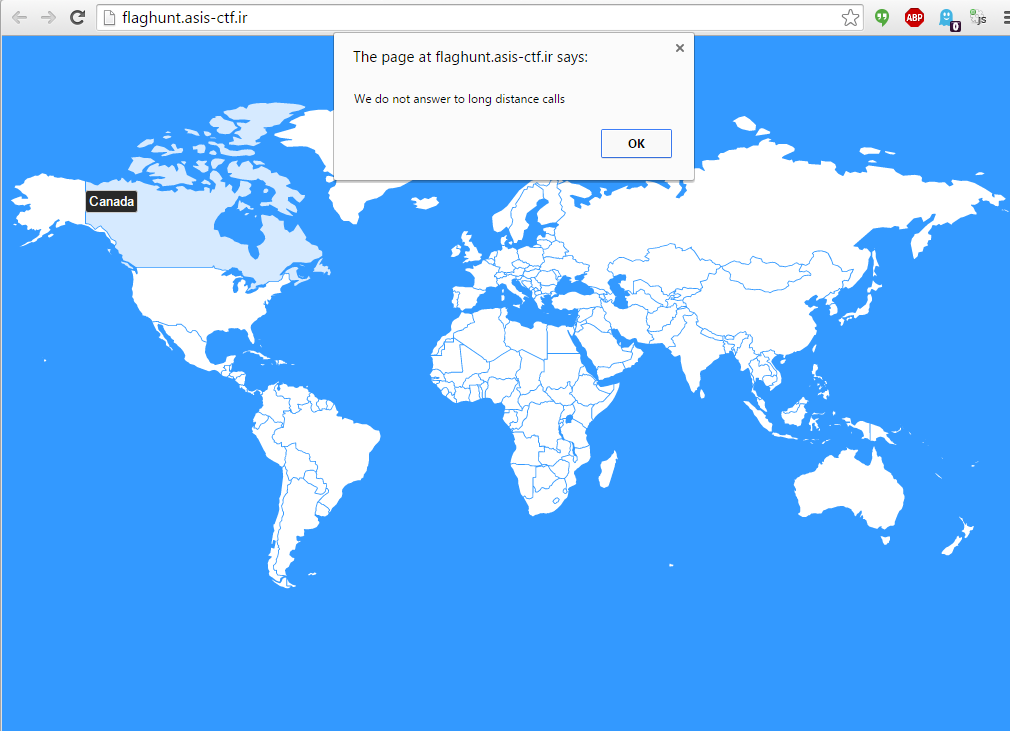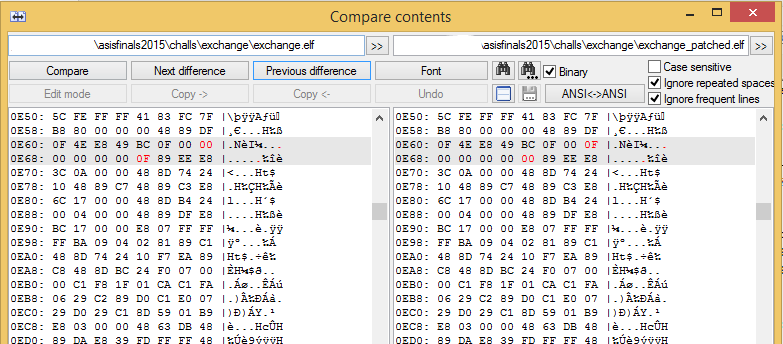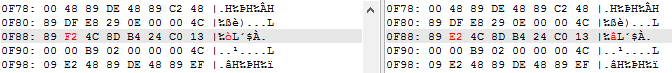# ASIS 2015 Finals: Flag Hunter (misc75)

A website showed the world map, clicking on your country (depending on your IP) gave you a part of the flag:Clicking on other country said you cannot do that:Solution was: using a few proxies, TOR, Hola VPN, etc the gather the 6 parts of the flag and then figure out in which order should we concatenate them (from the 6! = 720 possibilites). We know that the SHA256(SHA256(“ASIS{…}”)) hash should match with the hash of the real flag (which was used by the ASIS scoreboard in a client-side javascript code).

Usually on every ASIS CTF we have to use this trick, so if you haven’t know it yet, then don’t forget for next year. :)

# ASIS 2015 Finals: Fake (rev150)

Fake was a fairly simple binary. You had to supply a 64 bit integer in decimal form as argv and it multiplied with different values, shifted some and printed out as ASCII.

As we could suspect that the output is the flag (it was 40 bytes long instead of the 38 byte + null terminating zero), and the flag is starting with “ASIS{“ + 3 hex digit (0-9a-f), we would easily bruteforce the solution as there are only 16**3 = 4096 possibilities.

The only problem we had to solve is it multiplied two 64 bit integers, which caused overflow. So when we calculated the input value, we had to solve a very basic congruence.

This C# snippet generated every possible inputs:

Testing them was done by the following bash script:

And grepping the result gave me only valid flag:

# ASIS 2015 Finals: Exchange (rev200)

Exchange is a 200 pts reversing challenge.

It “encrypts” the flag and saves the result to the flag_encrypted file, which was given to us.

After reversing the code, I found out what is the purpose of different functions:

0x18B0 - int* asciiToBigInt(char* input, int input): converts an ASCII string (right padded with \x00 bytes to 128 len) to an internal representation (I call it “bigInt”) where every base 10 digit is stored as a 4 byte int containing byte 0x00 - 0x09. The data is in little endian order. So the last digit is the first integer.

For example if the input is “A”, then it is padded to “A”+”\x00”*127, which can be represented as big integer as:

So the internal representation will be as integer: 0,4,8,9,…,4,6,5,4. As bytes: 00000000 04000000 0800000000 0900000000 …

0x25F0 - void bigIntToDecStr(int input, char *outBuf): converts internal representation to decimal number string: “45644…59840” 0x276C - void bigIntToHex(int *a1, char *a2): converts internal representation to hex string: “410000…000000” 0x1780 - int decStrToBigInt(char inputDecStr): converts decimal number string to internal representation: “45644…59840” => 0,4,8,9,…,4,6,5,4 0x0f82 - void bigIntMultiply(int *output, int *input1, int *input2): output = input1 * input2 for bigints 0x21a0 - void bigIntAdd(int *result, int *a, int *b): result = a + b for bigints 0x1730 - __int64 hexCharToNum(int a1): converts one hex character to numerical representation: “0” -> 0, “A” -> 10, “F” -> 15 0x2540 - void divideBy2(int *input): input /= 2 for bigints 0x13B0 - int algoAvg(int *input1, int *input2, __int64 roundNum): this is the main magic method, can be summarized as this:

As the round number was too much and it would be days to run, I patched the binary and lowered the round from 0x0f0000000000000f to 0x0f000f which should give me almost the correct result.Also it OR-ed with small random values, which did not cause significant result, but could make my debugging harder, so I patched those out as well.I found out quickly that the result is (1input1 + 2input2)/3, but it calculates it a much slower way.

Knowing these functions the main method can be summarized as this:

• read flag as first argument (argv)
• converts the flag to a decimal number string => flagStr
• selects a random value between 1 and 127
• chunks the flagStr into two parts, the length of the first part is the previously generated random value
• calculates avg1 = algoAvg(part1, part2) = (part1 + 2*part2) / 3
• calculates avg2 = algoAvg(part2, 2part1) = (part2 + 4part1) / 3
• converts avg1 and avg2 to hex and writes them out to flag_encrypted file

Although I don’t understand exactly but in the cases I tested the two parts are separated by the 0x00, 0x01, 0x02 byte series.

So decrypting the flag can be done by:

• splitting the flag_enc file into two parts (avg1 and avg2), the parts are separated by 0x000102
• calculating (equations are determined by doing some basic math)
• input2 = (4 * avg1 - avg2) * 3 / 7
• input1 = 3 * avg1 - 2 * input2;
• joining the two parts* (as decimal strings)
• converting the big integer to ASCII

• I had to bruteforce a little as the input1’s last digits were not correct, so I tried to add 0..255 to input1 before joining the strings and doing this until I found “ASIS{“

The plaintext was:

# ASIS 2015 Finals: Example flag (trivia1)

This challenge was solved by and the write up was written by one of my teammates, nguyen

=> converts back from hex ‘476f20676f6f2e676c2f67776a625466’ == Go goo.gl/gwjbTf

It is a short link to http://0bin.asis.io/paste/wYO8nWC1#Fef7-CVtrDG7NIb0e1W77+t2jtMF8GSbVHuo6Ajm5RQ that has content:

# ASIS 2015 Finals: Calm down (trivia75)

We got a big file with a lot of flag-like stuff:

Running a grep solved the challenge (-a: text mode, -E: extended grep):